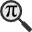## General

Display information for equation id:math.22190.22 on revision:22190

* Page found: Coupling from the past (eq math.22190.22)

(force rerendering)

Cannot find the equation data in the database. Fetching from revision text.

Occurrences on the following pages:

Hash: 4f08e3dba63dc6d40b22952c7a9dac6d

TeX (original user input):

\pi


TeX (checked):

\pi


### LaTeXML (experimental; uses MathML) rendering

MathML (561 B / 254 B) :${\displaystyle\pi}$
<math xmlns="http://www.w3.org/1998/Math/MathML" id="p1.1.m1.1" class="ltx_Math" alttext="{\displaystyle\pi}" display="inline" xref="p1.1.m1.1.cmml">
<semantics id="p1.1.m1.1a" xref="p1.1.m1.1.cmml">
<mi id="p1.1.m1.1.1" xref="p1.1.m1.1.1.cmml">π</mi>
<annotation-xml encoding="MathML-Content" id="p1.1.m1.1.cmml" xref="p1.1.m1.1">
<ci id="p1.1.m1.1.1.cmml" xref="p1.1.m1.1.1">π</ci>
</annotation-xml>
<annotation encoding="application/x-tex" id="p1.1.m1.1b" xref="p1.1.m1.1.cmml">{\displaystyle\pi}</annotation>
</semantics>
[/itex]


SVG (1.046 KB / 644 B) :

### MathML with SVG or PNG fallback (recommended for modern browsers and accessibility tools) rendering

SVG image empty. Force Re-Rendering

SVG (0 B / 8 B) :

PNG (0 B / 8 B) :

$\pi$## Translations to Computer Algebra Systems

### Translation to Maple

In Maple: pi

\pi: Could be the ratio of a circle's circumference to its diameter == Archimedes' constant.

But it is also a Greek letter. Be aware, that this program translated the letter as a normal Greek letter and not as a constant!

Use the DLMF-Macro \cpi to translate \pi as a constant.


### Translation to Mathematica

In Mathematica: \[Pi]

\pi: Could be the ratio of a circle's circumference to its diameter == Archimedes' constant.

But it is also a Greek letter. Be aware, that this program translated the letter as a normal Greek letter and not as a constant!

Use the DLMF-Macro \cpi to translate \pi as a constant.


## Similar pages

Calculated based on the variables occurring on the entire Coupling from the past page

## Identifiers

• $\pi$### MathML observations

0results

0results

no statistics present please run the maintenance script ExtractFeatures.php

0 results

0 results